# Ensemble: Scikit-learn and Keras, Part2: Regressors

Hi All,
In the last blog I went over how to use ensemble methods with both Scikit-learn models and Keras model for classification.

In this blog I want to show you how to do this for regression problems. I thought it wouldn’t be much useful till I was toiling around with the regression problems esp. using different ensemble methods that I realized this blog could be useful esp. to look at the different ensemble methods and see which one is the best.

Before we dive in a quick reminder of what ensemble methods are. Imagine you work for a big tech firm that specializes in machine learning and there is a very important task that you want to done perfectly. Ideally, as this is a big tech firm, there would be more than just one or two machine learning engineers working on this, you would have teams of engineers working on it.

Now imagine there are say five best models that work really well, now you can choose one of them and put in production or if you are a little risk averse as I am you would rather use all five models and take an average of them. Remember the premise was that this is an important task and you can run these models in parallel if you need to so the overhead of running five models is not that critical if this improves accuracy.

As you might have guessed this is what ensemble does. It takes an (weighted) average of a few models to come up the final answer and since this is running based on more than one model the accuracy usually improves. If this all sounds familiar, I apologize for the repetition but I really want to hammer in the concept of average different models to improve accuracy.

We are going to use both Scikit learn based models and deep neural network models from Keras. As always we follow the below steps to get this done.

1. Dataset: Load the data set, do some feature engineering if needed.
2. Build Models: Build a TensorFlow model with various layers.
3. Fit Models: Here we finally train the model using the training data and get some metrics.
4. Evaluate Models: We check our model performance on the validation data.

Dataset:
We use the inbuilt and readily available Boston housing dataset from Scikit learn.

First, let’s look at how to load data. Since this is an in-built data set from Scikit learn we just call the function from Scikit-learn. You can read more about the data from here.

`#Usual Importsimport pandas as pdfrom sklearn.linear_model import LinearRegressionfrom sklearn.ensemble import RandomForestRegressorfrom sklearn.experimental import enable_hist_gradient_boostingfrom sklearn.ensemble import VotingRegressor,GradientBoostingRegressor,HistGradientBoostingRegressor,StackingRegressorfrom sklearn.model_selection import train_test_splitfrom sklearn.svm import SVRfrom sklearn.metrics import mean_squared_error,accuracy_scorefrom sklearn.ensemble import AdaBoostRegressor,BaggingRegressor,ExtraTreesRegressorfrom xgboost import XGBRegressorimport tensorflow as tfimport tensorflow.keras as kerasfrom tensorflow.keras.layers import Dense,Dropoutfrom tensorflow.keras.models import Sequentialfrom keras.metrics import RootMeanSquaredErrorimport warningswarnings.filterwarnings('ignore')#load the Boston housing datasetfrom sklearn.datasets import load_bostonboston_dataset = load_boston()X = pd.DataFrame(boston_dataset.data,               columns=boston_dataset.feature_names)y = boston_dataset.target`

Build Models:

`#Scikit-learn Modelslin_reg= LinearRegression()rnd_reg =RandomForestRegressor(n_estimators=100, random_state=42)svr_reg = SVR(gamma="scale")#Keras Modeldef build_nn():    model= Sequential(                [Dense(512,activation='selu',input_shape=),                 Dense(256,activation='selu'),                 Dropout(0.2),                 Dense(128,activation='selu'),                 Dense(64,activation='selu'),                 Dense(1)    ])    model.compile(optimizer='adam',              loss='mean_squared_error',              metrics=['RootMeanSquaredError'])    return model`

Till now there is nothing new as we plainly building models from Scikit-learn and Keras. Here comes the magic line that changes everything.

`keras_reg = tf.keras.wrappers.scikit_learn.KerasRegressor(                build_nn,epochs=1000,verbose=False)`

This one line wrapper call converts the Keras model into a Scikit-learn model that can be used for Hyperparameter tuning using grid search, Random search etc. but it can also be used, as you guessed it, for ensemble methods.

Since this is a regressor we need one additional line to get this working.

`keras_reg._estimator_type = "regressor"#https://stackoverflow.com/questions/59897096/votingclassifier-with-pipelines-as-estimators/59915844#59915844`

Finally we define the voting regressor using the below code.

`voting_reg = VotingRegressor(             estimators=[('lr', lin_reg),                          ('rf', rnd_reg),                         ('svr', svr_reg),                         ('Dense',keras_reg)])`

This is pretty much what we did in the last blog but modified for regression. This week I want to go further and use “Stacking” ensemble method.

Stacking is an ensemble method where instead of taking a weighted average, we just train a model to perform the final aggregation. Since our problem at hand is a regression one, we can use any of the regressors available from Scikit learn. What’s more interesting we can even use XGBoost regressor to be our final regressor. Here is how I did.

`#Default: RidgeCVst_reg=StackingRegressor(        estimators=[('lr', lin_reg),                    ('rf', rnd_reg),                    ('svr', svr_reg),                    ('Dense',keras_reg)])`

Fit Models:

Now that we have all our regressors setup and ready, lets fit the models.

`for reg in (lin_reg, rnd_reg, svr_reg,keras,voting_reg,st_reg):    reg.fit(X_train, y_train)    y_pred = reg.predict(X_test)    print(reg.__class__.__name__,          mean_squared_error(y_test, y_pred,squared=False))    print('R2 score: {:.2f}'.format(r2_score(y_test, y_pred)))`

Evaluate Models:
Now the final step to see how these models performed.

Default: Stacking with no final estimator, ie default RidgeCV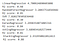RidgeCV

GradientBoostingRegressor:
Stacking with final estimator GradientBoostingRegressor.

`st_reg=StackingRegressor(                        estimators=[('lr', lin_reg),                                    ('rf', rnd_reg),                                    ('svr', svr_reg),                                     ('Dense',keras)],                       final_estimator=                         GradientBoostingRegressor(random_state=42))`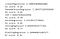GradientBoostingRegressor

ExtraTreesRegressor:
Stacking with final estimator ExtraTreesRegressor.

`st_reg=StackingRegressor(                        estimators=[('lr', lin_reg),                                    ('rf', rnd_reg),                                    ('svr', svr_reg),                                     ('Dense',keras)],                        final_estimator=                               ExtraTreesRegressor(random_state=42))`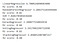ExtraTreesRegressor

HistGradientBoostingRegressor:
Stacking with final estimator HistGradientBoostingRegressor.

`st_reg=StackingRegressor(                        estimators=[('lr', lin_reg),                                    ('rf', rnd_reg),                                    ('svr', svr_reg),                                     ('Dense',keras)],               final_estimator=                     HistGradientBoostingRegressor(random_state=42))`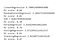HistGradientBoostingRegressor

XGBoost:
Finally using XGBoost as the regressor and the final regressor

`import xgboost as xgbxgb_reg=xgb.XGBRegressor(random_state=42)st_reg=StackingRegressor(                        estimators=[('lr', lin_reg),                                    ('rf', rnd_reg),                                    ('svr', svr_reg),                                    ('xgb', xgb_reg),                                    ('Dense',keras)],                     final_estimator=                                        XGBRegressor(random_state=42))`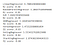XGBRegressor

All of this code is available here at my Github repository.

Finally, I want to take this opportunity to thank Aurelien Geron for his excellent book “Hands-on Machine Learning with Scikit-Learn, Keras & Tensorflow”. Hope you would find this blog useful.

Good luck !!!

References:

Hands-On Machine Learning with Scikit-Learn, Keras & TensorFlow by Aurelien Geron